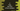# C program to find the GCD of two user given numbers in 2 different ways## C program to find the GCD of two user given numbers:

In this tutorial, we will learn how to find the GCD of two numbers in C. GCD stands for Greatest common divisor and it is also known as the greatest common factor (gcf) or highest common factor (hcf).

### Example of GCD:

Let’s consider two numbers : 200 and 560. Divisors of 200 are :

``````1
2
4
5
8
10
20
25
40
50
100``````

And, divisors of 560 :

``````1
2
4
5
7
8
10
14
16
20
28
35
40
56
70
80
112
140
280``````

The greatest common number in these two lists is 40. This is GCD.

### How to find out GCD of two numbers:

To find out the GCD of two numbers, we can find the divisors of both numbers separately and find out the greatest common divisors from the two set. Another way to do that is by using Euclidean Algorithm. Euclidean Algorithm says that if we subtract the smaller number from the bigger number, the GCD doesn’t change. If we keep subtracting the smaller number from the bigger number, we will get the GCD at end.

For 560 and 200,

``````numbers 560,200 : 560 - 200 = 360
numbers 360,200 : 360 - 200 = 160
numbers 160,200 : 200 - 160 = 40
numbers 160,40 : 160 - 40 = 120
numbers 120,40 : 120 - 40 = 80
numbers 80,40 : 80 - 40 = 40
numbers 40,40``````

So 40 is the GCD.

### C program to calculate GCD :

``````#include <stdio.h>

int findGCD(int first, int second)
{
if (first == 0 || second == 0)
{
return first == 0 ? second : first;
}

return first > second ? findGCD(first - second, second) : findGCD(first, second - first);
}

int main()
{
int firstNo, secondNo;

printf("Enter the first number :");
scanf("%d", &firstNo);

printf("Enter the second number :");
scanf("%d", &secondNo);

int gcd = findGCD(firstNo, secondNo);

printf("GCD : %d", gcd);
}``````

### Sample output :

``````Enter the first number :560
Enter the second number :200
GCD : 40``````

## Method 2:

Another efficient way to use Euclidean Algorithm is by using division. If we keep dividing the bigger number by the smaller number, we will get the GCD if one becomes zero.

``````#include <stdio.h>

int findGCD(int first, int second)
{
if(second == 0){
return first;
}

return findGCD(second, first%second);
}

int main()
{
int firstNo, secondNo;

printf("Enter the first number :");
scanf("%d", &firstNo);

printf("Enter the second number :");
scanf("%d", &secondNo);

int gcd = findGCD(firstNo, secondNo);

printf("GCD : %d", gcd);
}``````